# Effect of Room’s Temperature and Electrode Gap on Current of Negative Corona Discharge in Rod-Plane Electrode Configuration

Effect of Room’s Temperature and Electrode Gap on Current of Negative Corona Discharge in Rod-Plane Electrode Configuration

El Hanafi OuatahSoufiane Megherfi Boukhalfa Bendahmane Youcef Zebboudj

Laboratoire de Génie Electrique de Bejaia, Université de Bejaia, Bejaia 06000, Algeria

Corresponding Author Email:
elhanafi.ouatah@univ-bejaia.dz
Page:
298-304
|
DOI:
https://doi.org/10.18280/mmep.090202
31 January 2022
|
Accepted:
6 April 2022
|
Published:
28 April 2022
| Citation

OPEN ACCESS

Abstract:

The stable corona discharge is widely used in filtration and electrostatic separation in recent years, and several models have been used by researchers to analyze one of its most important properties which is the current-voltage characteristic. The aim of this paper is to investigate the influence of ambient temperature and electrodes’ gap on negative DC discharge using rod-plane geometry, and the Townsend formula was found to be most appropriate model (I=K.V.(V-V0)). The experimental results show that for the same voltage level applied to the high voltage electrode, the discharge current rises with increasing temperature and decreases as the electrodes’ gap increases. Using curve ﬁtting, it was proven that the geometric factor K is proportional to temperature and to the power of the distance between electrodes independently, and the threshold voltage V0 is proportional to the product of the temperature reciprocal and the power of the inter-electrode spacing. From these results, a new modified Townsend formula by introducing the air temperature and the distance between electrodes is proposed to calculate the discharge current with an accuracy of ±10%.

Keywords:

corona discharge, current-voltage characteristic, ambient temperature, Townsend empirical formula

1. Introduction

The corona discharge is used in numerous industrial applications, such as electrostatic separation and filtration [1-4], neutralization of the electric charge present on the surface of dielectric materials , surface treatment  and electrostatic painting . These multiple applications encourage us to study this type of discharge and the factors influencing its parameters.

A corona discharge is a self-sustained electric gas discharge, where the electric field confines the primary ionization processes to regions near the high electric field electrode. A direct current (DC) corona discharge is called positive or negative, depending on the polarity of the high field electrode and the negative corona occurs only in electronegative gases . For both polarities, the electrostatic forces linked to the movement of charges under the effect of the electric field induce an airflow called ionic wind or corona wind .

In negative corona discharge, when the voltage applied to the corona electrode increases, different phases of discharge appear. Goldman and Goldman  have classified the currents discharge of these phases to (a) auto-stabilization, (b) regular pulses and (c) continuous current discharge. The pulses observed in the phase (b) are named Trichel pulses  and are regular with very short rise times (<1.3ns) and separated by longer inter-pulse periods (tens of µs) [12, 13]. These pulses occur in electronegative gases only and they are very regular in oxygen and air, but they are more irregular in some other gases, such us the SF6.

Several researches have been conducted to express the mean current of the Trichel pulses as a function of the applied voltage. Usually, the current-voltage characteristic of negative or positive corona discharge is governed by the Towsend empirical formula: I=K.V.(V-V0), where K is a coefficient that depends on electrode configuration, charge carrier mobility, temperature, pressure and humidity, and V0 represents the inception voltage of the corona effect. Originally, this formula was found for coaxial wire-cylinder geometry [14, 15]. After that, it was shown that this empirical formula can be applied for other geometry, such as wires-plane [16-22], rod-plane [23, 24], wire-cylinder [25, 26] and rod-grid . In 1986, Ferreira et al.  said that negative corona discharge’s current in rod-plane electrode system can be expressed by A(V-V0)2. Then, in 2008, Meng et al.  generalized this model in the form of A'(V-V0)n. Where, A and A’ are factors which depend on the geometric parameters of the electrodes and the physical parameters of air and V0 is the threshold or inception voltage of corona discharge.

The objective of this work is the experimental study of the negative corona discharge in rod-plane geometry and the influence of the room’s temperature and the electrodes’ gap on its parameters and an empirical formula based on that Townsend is developed. The characteristics of the current-voltage measurements are analyzed by the empirical formula of Townsend, this model has been generalized by introducing the temperature and the electrodes’ gap to calculate the discharge current with an acceptable precision.

2. Experimental Procedure

The negative corona discharge reactor used in these experiments consisted of rod-plane electrodes’ system, as shown schematically in Figure 1.

The negative DC voltage supplied by the high voltage source (1) (± 140 kV, 80 mA) measured using the resistive bridge (2) and the peak voltmeter (MU11) is applied to the corona electrode of the discharge cell (3). This one consists of: a high-voltage electrode in form of tip with small curvature radius (r ≈ 40 µm) which is fixed with an insulating support and a ground electrode in form of circular plane with radius of 360 mm. Moreover, a cylindrical guard electrode is used to protect the system from external electromagnetic ﬁeld. To study the temperature’s influence on the corona discharge parameters, the discharge cell is placed inside the temperature-controlled oven (4) of volume (800 * 600 * 480) mm3 which enables to reach a temperature of 330℃.

The installation elements under high voltage are separated from the manipulator and the low voltage measuring devices by the protective grid (5).

In order to characterize the corona discharge, the installation is equipped with measuring devices (6). In this case, the DC high voltage is measured by the peak voltmeter MU11 and the discharge current by the micro-ammeter. As it is known, the physical parameters of the air greatly influence the corona discharge, it is then necessary to record systematically these parameters before each test. The mercury barometer is used for pressure with a measuring range 75 to 1030 hPa and a reading accuracy of 1 hPa, the mercury thermometer for the temperature with a measuring range of 0 to 350℃ and a reading accuracy of 0.5℃ and the hair hygrometer for humidity with a measuring range 0 to 100% and a reading accuracy +/- 3%.

## 1.pngFigure 1. Scheme of the experimental device

3. Results and Discussions

3.1 Current-voltage characteristic

The gradual increase of applied voltage was made manually from 0 to V1 such that 0<V0<V1, where V0 is the inception voltage of the corona effect and V1 is a voltage much lower than the breakdown voltage Vc. A safety margin of 8 to 10 kV separates voltages V1 and Vc. The simultaneous measurement of the applied voltage and the discharge current allowed us to draw the current-voltage (I-V) characteristic of the discharge. The variation of discharge current as a function of applied voltage is shown in Figure 2. These results are obtained with HV electrode of curvature radius about r=40μm, the inter-electrode spacing is d=5cm, with atmospheric conditions of the order of: temperature T=303K, pressure P=1017hPa and relative humidity Hr=60%.

## 2.png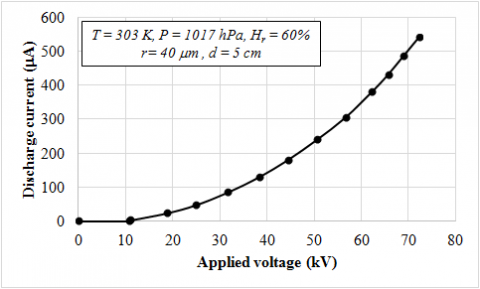Figure 2. Current-voltage characteristic of rod-plane electrode’s system

According to Figure 2, the discharge current increases with non-linear manner as a function of the rise in the applied voltage. After analyzing our results by different models, namely the model of Townsend [14, 15], the model of Ferreira  and the model of Meng , it’s to be noted that the most suitable model is that of Townsend which is governed by Eq. (1).

$I=K \cdot V \cdot\left(V-V_{0}\right)$                     (1)

To determine the parameters of the Eq. (1) (K and V0), the ratio (I/V) was represented as a function of V, such as:

$I / V=K \cdot\left(V-V_{0}\right)$                     (2)

where: I is the corona discharge current, V the applied voltage, V0 the inception voltage of corona phenomena and K is a constant depending on electrodes’ geometry and ion mobility.

3.2 Effect of electrodes’ gap on I-V characteristic

Figure 3 shows the I-V characteristics of negative corona discharge for different electrodes gaps with the atmospheric conditions given in the same figure.

## 3.png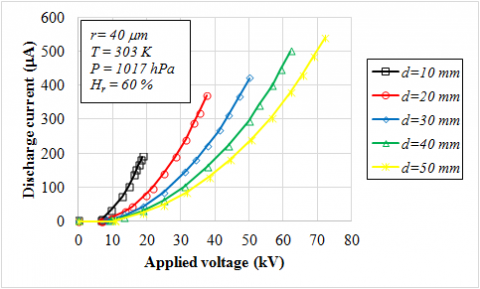Figure 3. Effect of electrodes’ gap on I-V characteristic of negative corona discharge

According to the Figure 3, the I-V characteristics are strongly affected by the electrode gap. At a given applied voltage V, the corona current decreases signiﬁcantly with the electrode gap d.

It can be also noted that the elevation of the electrode gap increases the operating voltage range between the breakdown and the inception voltages, also, a wide range of stable corona can be obtained because of the breakdown voltage rise.

Figure 4 illustrates the discharge current at different electrodes gaps with maintaining the voltage applied to the active electrode at 18.5 kV.

## 4.png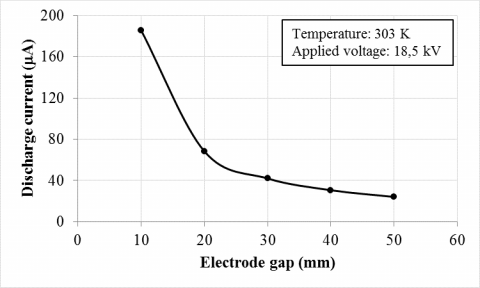Figure 4. Corona current with variation of electrode gap

The corona current decreases as the electrodes’ gap increases, e.g., it reduces from 185.5 µA to 24 µA when the electrodes’ gap rises from 10 mm to 50 mm. The external electric ﬁeld of the small electrodes’ gaps is greater than that of the big electrodes’ gaps under the same applied voltage, thus indicates higher ionization coefficient for the small electrode’s gaps than for the big ones. The resulting space charge from the large number of negative ions produced in this case increases the local electric field . As results, the increase of the electrodes’ gap causes the reduction of the total electric field, which implies a decrease in the discharge current.

In order to study the effect of the space between electrodes on the parameters of Eq. (1), the ratio I/V as function of voltage V is plotted in Figure 5 for different electrodes’ gaps at an ambient temperature of 303 K.

## 5.png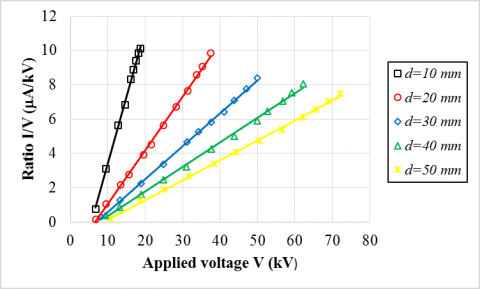Figure 5. Linear fits of the characteristic (I/V, V) with different electrodes’ gap

## 6.png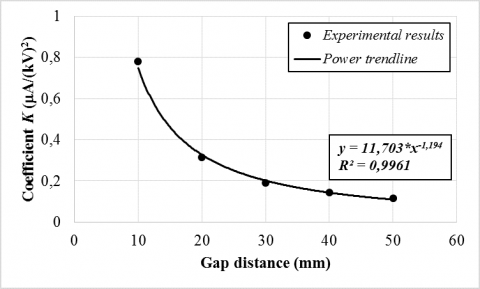Figure 6. Dependence of the coefficient K with electrodes’ gap

For all cases of electrodes’ gap, a linear dependence of I /V versus V is clearly shown in Figure 5 and the fits of these curves gave straight lines of equations: y=a+b*x. The slope b represents the geometric coefficient K of the Eq. (1) and the y-intercept a represents (–K.V0).

The variation of the coefficient K and the inception voltage V0 with the electrodes’ gap can be obtained by the characteristics' (I/V, V) fitting with the least squares method. The fits' results are plotted in Figure 6 and Figure 7.

According to Figure 6, the coefficient K decays as the gap distance d increases. In addition to that, curve fitting via least squares approach enabled the determination of the mathematical relation between d and K. One can notice that the resulting power trendline has an exponent coefficient of (-1.194) and a linear regression coefficient of R2=0.9961. Hence, Townsend’s formula coefficient K is inversely proportional to (d1.194). A similar result has already been found in rod-grid electrode system . To this end, the dependence of K on d is given as:

$K \propto d^{-1.194}$                (3)

## 7.png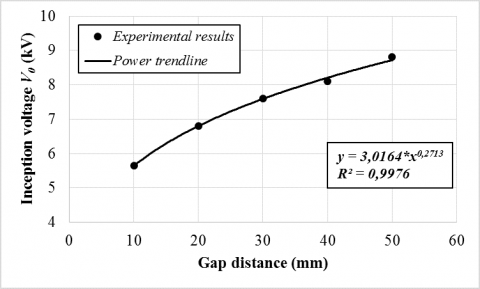Figure 7. Inception voltage as a function of electrodes’ gap

Figure 7 shows that the inception voltage of corona discharge of Eq. (1) grows non-linearly with the inter-electrode spacing d. It was already observed that the corona onset voltage is proportional to the electrodes’ gap power [28, 30]. The power curve fitting is used to determine the variation's law of V0 as a function of d and it was found that the trendline has an exponent coefficient of about (0.2713) with an excellent coefficient of determination (R2=0.9976). The resultant dependence of V0 with the distance d is written as:

$V_{0} \propto d^{0.2713}$                 (4)

3.3 Temperature effect on I-V characteristic

The corona discharge current is a function of physical and geometrical parameters. When the electrode system is chosen with fixed inter-electrode spacing and the medium humidity and pressure are monitored, the discharge current depends only the ambient temperature.

Figure 8 represents the I-V characteristics of the rod-plane conﬁguration at temperatures ranging from 303 K to 363 K with the electrodes’ gap of 50 mm. This variation is quadratic and in good agreement with the classical model of current-voltage characteristic formulated by Townsend. It is to be noted that the negative corona current rises with the room’s temperature elevation. It grows from 431 µA at 303 K to 561 µA at 363 K with maintaining the intensity of applied voltage to the HV electrode at 65 kV.

## 8.png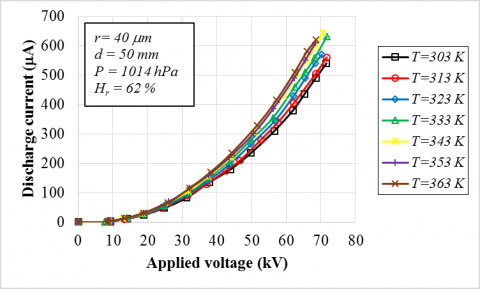Figure 8. Temperature effect on I-V characteristic of negative corona discharge

Figure 9 depicts the behavior of the corona discharge at room and at high temperatures.

## 9.pngFigure 9. Corona discharge’s behavior

Comparing the two diagrams, it results that the number of negative ions collected at the grounded plate electrode goes up significantly at high temperatures. When the air between the electrodes is hot, the mean free path becomes long and the ionization zone widens, thus increasing the number of electrons produced in the ionization zone. Besides, additional ions are created by attachment of the extra electrons produced in the ionization zone. It results that the free electrons produced during the initial gas ionization process are accelerated between the high-voltage electrode and the grounded plate by the external electric field. Also, the electronic energy increases . Therefore, Townsend's first ionization coefficient α, denoting the electrons’ number produced by an electron per unit length of path in the direction of field, which depends on electronic energy, rises with temperature elevation. As a result, and according to the Eq. (5), the discharge current rises with the increase of ionization coefficient.

$i=\frac{i_{0} e x p^{\alpha d}}{1-\gamma\left(e x p^{\alpha d}-1\right)}$                    (5)

Moreover, at very high temperatures, in addition to the ion current, an electron current must be considered [32, 33].

In order to determine the temperature’s influence on the parameters of Eq. (1), the results shown in Figure 8 are used to plot the I /V ratio as a function of the voltage V applied to the corona electrode (Figure 10). A linear dependence of I/V versus V is observed at all temperature. The linear fits of these straight lines will allow us to determine the two parameters K and V0 of the Townsend formula at each temperature degree.

## 10.png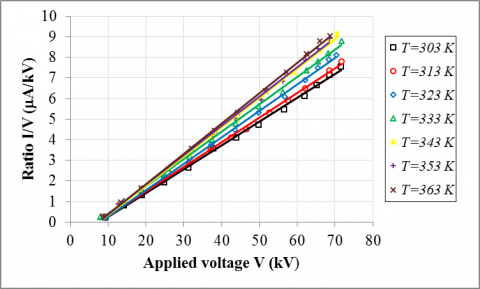Figure 10. Linear fits of the characteristic (I/V, V) at different temperature degrees

Figure 11 and Figure 12 indicate the dependence of respectively the coefficient K with temperature and the voltage V0 with the reciprocal of temperature.

## 11.png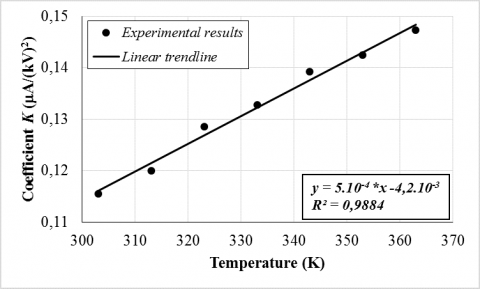Figure 11. Variation of the coefficient K in the Townsend formula with temperature

It results from Figure 11 that the temperature dependence of the coefficient K is linear. In this case the straight-line lengthening intersects the temperature axis at T0 = 84 K which corresponds to the critical temperature of air. At this temperature, the ion mobility is zero. Therefore, the coefficient K is equal to zero because it’s proportional to the ion mobility. As a result, the dependence of the coefficient K on the temperature T can be given by :

$K \propto(T-88)$                    (6)

In his theoretical study, Uhm  affirmed that the corona inception voltage is inversely proportional to the ambient temperature in a coaxial wire-cylinder electrode system. Based on this analysis, the dependence of V0on 1/T is plotted in Figure 12.

## 12.pngFigure 12. Variation of the inception voltage V0 in the Townsend formula with the reciprocal temperature

Figure 12 displays that corona threshold voltage V0 rises linearly with the reciprocal temperature (1/T). Consequently, the temperature dependence of V0 can be expressed as:

$V_{0} \propto T^{-1}$                   (7)

4. New Empirical Formula

In rod-plane electrode system, the most suitable model for current-voltage characteristics is that of Townsend. According to the experimental results presented above, it has been proved that when the electrode system is chosen and the environment conditions have been ﬁxed, the coefficient K is proportional to d-1.194 and (T-84), also, the inception voltage V0 is proportional to d0,2713 and T-1. From these results, it is to be noted that the Townsend model governing the current-voltage characteristic can be generalized in a new form described by:

$I=C_{1} \cdot \frac{T-84}{d^{1,194}} \cdot V\left(V-C_{2} \cdot \frac{d^{0,2713}}{T}\right)$                     (8)

So:

$I / V=C_{1} \cdot \frac{T-84}{d^{1,194}} \cdot\left(V-C_{2} \cdot \frac{d^{0,2713}}{T}\right)$                     (9)

where: C1 and C2 are coefﬁcients depending on electrodes’ geometry, the humidity and the pressure values. Moreover, the coefficients C1 and C2 are determined by fitting via least squares approach of results plotted in Figure 5 and Figure 10 with Eq. (9). The resultant values are:

$C_{1}=0,05318 \pm 0,0049 \mu \mathrm{A} \mathrm{mm}{ }^{1,194} /\left((\mathrm{kV})^{2} \mathrm{~K}\right)$

$C_{2}=978,56 \pm 21,29 \mathrm{kV} \mathrm{K} / \mathrm{mm}^{0,2713}$

By replacing the two constants C1 and C2 in Eq. (8), the final form of the modified Townsend equation is obtained:

$I=0,05318 \frac{T-84}{d^{1,194}} V\left(V-978,56 \frac{d^{0,2713}}{T}\right)$                   (10)

In Figure 13, it is plotted with the same conditions of temperature and electrodes’ gap the calculated current with the Eq. (10) as a function of the measured discharge current.

Ideally, all the points of the curve must belong to the straight line of equation y=x (solid line). Figure 13 shows that all the points are contained in the zone delimited by the dashed lines which correspond to the acceptable error margin of ±10%. This result allows us to conclude that with the electrodes’ geometry and the experimental conditions described in the previous sections, the current of negative corona discharge can be calculated with Eq. (10).

## 13.png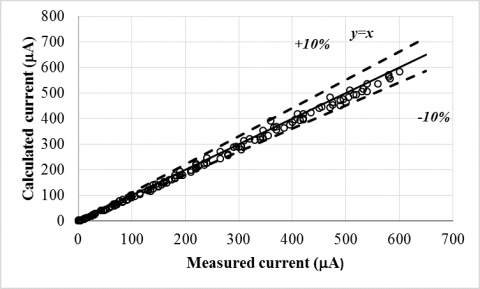Figure 13. Comparison of measured corona discharge currents with those calculated by the new empirical formula

5. Conclusion

In this paper, the effect of temperature degree and electrodes’ gap on the current of negative corona discharge in rod-plane electrode system has been investigated experimentally. It was noticed that the discharge current decreases significantly with the electrodes’ gap widening and increases slightly with the ambient temperature elevation.

All current-voltage characteristics have been analyzed with the classical Townsend formula and the results are very satisfactory. It has been shown experimentally that the coefficient K of the Townsend formula rises linearly with room temperature and diminishes with the power of inter-electrode spacing, while the inception voltage of corona effect decreases with the air temperature and increases with the power of the electrodes’ gap.

In this work, the mathematical processing of experimental data allowed us to generalize the empirical Townsend formula by introducing temperature and distance between electrodes.

Acknowledgment

The authors would like to express their deepest gratitude to all the members of the high voltage laboratory of Bejaia and the General Directorate of Scientific Research and Technological Development (DGRSDT) of the Higher Education and Scientific Research Algerian Ministry as a sponsoring institution.

References

 Kouidri, M.A., Mahi, D. (2018). Study and realization of an electrostatic precipitator device. Modelling. Measurement and Control C, 79(4): 235-241. http://dx.doi.org/10.18280/mmc_c.790415

 Mohanta, S.K., Dwari, R.K. (2020). Separation of the coal-quartz mixture using tribo-electrostatic separator: Effect of surface pretreatment. Advanced Powder Technology, 31(8): 3361-3371. http://dx.doi.org/10.1016/j.apt.2020.06.027

 Bedeković, G., Trbović, R. (2020). Electrostatic separation of aluminium from residue of electric cables recycling process. Waste Management, 108: 21-27. http://dx.doi.org/10.1016/j.wasman.2020.04.033

 Chen, Z. Tian, E., Mo, J. (2020). Removal of gaseous DiBP and DnBP by ionizer-assisted filtration with an external electrostatic field. Environmental Pollution, http://dx.doi.org/10.1016/j.envpol.2020.115591

 Messaoudène, A., Mekidèche, M.R., Bendahmane, B., Tabti, B., Dascalescu, L. (2020). Experimental study of controlled active neutralization of polypropylene films. Journal of Electrostatics, 103: 103407. http://dx.doi.org/10.1016/j.elstat.2019.103407

 Oudrhiri Hassani, F., Merbahi, N., Oushabi, A., Elfadili, M.H., Kammouni, A., Oueldna, N. (2020). Effects of corona discharge treatment on surface and mechanical properties of Aloe Vera fibers. Materials Today: Proceedings, 24: 46-51. https://dx.doi.org/10.1016/j.matpr.2019.07.527

 Joseph, R. (2009). Painting problem solver: Ensuring proper grounding when working with an electrostatic spray gun, Metal Finishing, 107(1): 47. https://dx.doi.org/10.1016/S0026-0576(09)80010-X

 Sigmond, R.S., Goldman, M. (1983). Electrical Breakdown and Discharges in Gases. Plenum Press, USA.

 Deng, J., Qu, H., Lin, J., Yu, G., Deng, Q. (2016). Analysis of the movement characteristics of corona winds during Needle-plate discharge. International Journal of Heat and Technology, 34(4): 574-580. https://dx.doi.org/10.18280/ijht.340404

 Goldman, M., Goldman, A. (1978). Gaseous Electronics, New York Academic Press, USA.

 Trichel, G.W. (1938). The mechanism of the negative point to plane corona near onset. Physical Review, 54(12): 1078-1084. http://dx.doi.org/10.1103/PhysRev.54.1078

 Soria-Hoyo, C., Pontiga, F., Castellanos, A. (2007). Particle-in-cell simulation of Trichel pulses in pure oxygen. Journal of Physics D: Applied Physics, 40(15): 4552. http://dx.doi.org/10.1088/0022-3727/40/15/027

 Ouatah, E., Megherfi, M., Haroun, K., Zebboudj, Y. (2013). Characteristics of partial discharge pulses propagation in shielded power cable. Electric Power Systems Research, 99: 38-44. http://dx.doi.org/10.1016/j.epsr.2013.01.012

 Townsend, J.S. (1915). Electricity in Gases. Oxford: Clarendon Press.

 Townsend, J.S. (1948). Electron in Gases. Hutchinson’s Scientific and Technical Publications.

 Cooperman, P. (1960). A theory for space-charge-limited currents with application to electrical precipitation. Transactions of the American Institute of Electrical Engineers, Part I: Communication and Electronics, 79(1): 47-50. http://dx.doi.org/10.1109/TCE.1960.6368541

 Cooperman, G. (1981). A new current-voltage relation for duct precipitators valid for low and high current densities. IEEE Transactions on Industry Applications, IA-17(2): 236-239. http://dx.doi.org/10.1109/TIA.1981.4503931

 Nouri, H., Zouzou, N., Moreau, E., Dascalescu, L., Zebboudj, Y. (2012). Effect of relative humidity on current–voltage characteristics of an electrostatic precipitator. Journal of Electrostatics, 70(1): 20-24. http://dx.doi.org/10.1016/j.elstat.2011.08.011

 Khaled, U., Eldein, A.Z. (2013). Experimental study of V-I characteristics of wire-plate electrostatic precipitators under clean air conditions. Journal of Electrostatics, 71(3): 228-234. https://dx.doi.org/10.1016/j.elstat.2012.12.023

 Aissou, M, Ait Said, H., Nouri, H., Zebboudj, Y. (2013). Analysis of current density and electric field beneath a bipolar DC wires-to-plane corona discharge in humid air. European Physical Journal Applied Physics, 61(3): 30803. http://dx.doi.org/10.1051/epjap/2013120172

 Ait Said, H. Nouri, H., Zebboudj, Y. (2014). Analysis of current-voltage characteristics in the wires-to-planes geometry during corona discharge. European Physical Journal Applied Physics, 67(3): 30802. http://dx.doi.org/10.1051/epjap/2014140089

 Aissou, M., Ait Said, H. Nouri, H., Zebboudj, Y. (2015). Effect of relative humidity on current-voltage characteristics of monopolar DC wire-to-plane system. Journal of Electrostatics, 76: 108-114. http://dx.doi.org/10.1016/j.elstat.2015.05.019

 Meng, X., Zhang, H., Zhu, J. (2008). A general empirical formula of current–voltage characteristics for point-to-plane geometry corona discharges. Journal of Physics D: Applied Physics, 41(6): 065209. http://dx.doi.org/10.1088/0022-3727/41/6/065209

 Moreau, E., Audier, P., Benard, N. (2018). Ionic wind produced by positive and negative corona discharges in air. Journal of Electrostatics, 93: 85-96. http://dx.doi.org/10.1016/j.elstat.2018.03.009

 Zebboudj, Y., Hartmann, G. (1999). Current and electric field measurements in coaxial system during the positive DC corona in humid air. European Physical Journal Applied Physics, 7(2): 167-176. http://dx.doi.org/10.1051/epjap:1999211

 Zebboudj, Y., Iken, R. (2000). Positive corona inception in HVDC configurations under variable air density and humidity conditions. European Physical Journal Applied Physics, 10(3): 211-218. http://dx.doi.org/10.1051/epjap:2000134

 Yamada, K. (2004). An empirical formula for negative corona discharge current in point-grid electrode geometry. Journal of Applied Physics, 96(5): 2472-2475. http://dx.doi.org/10.1063/1.1775301

 Ferreira, G.F.L., Oliveira, O.N., Giacometti, J.A. (1986). Point-to-plane corona: Current voltage characteristics for positive and negative polarity with evidence of an electronic component. Journal of Applied Physics, 59(9): 3045-3049. http://dx.doi.org/10.1063/1.336926

 Wang, W., Zhang, J., Liu, F., Liu, Y., Wang, Y. (2004). Study on density distribution of high-energy electrons in pulsed corona discharge. Vacuum, 73(3-4): 333-339. http://dx.doi.org/10.1016/j.vacuum.2003.12.047

 Giubbilini, P. (1988). The current-voltage characteristics of point-to-ring corona. Journal of Applied Physics, 64(7): 3730-3732. http://dx.doi.org/10.1063/1.341368

 Peek, F.W. (1929). Dielectric Phenomena in High-Voltage Engineering. McGraw-Hill Book Company, Inc.

 Yan, P., Zheng, C., Xiao, G., Xu, X., Gao, X., Luo, Z., Cen, K. (2015). Characteristics of negative DC corona discharge in a wire-plate configuration at high temperatures. Separation and Purification Technology, 139: 5-13. http://dx.doi.org/10.1016/j.seppur.2014.10.026

 Yan, P., Zheng, C., Zhu, W., Xu, X., Gao, X., Luo, Z., Ni, M., Cen, K. (2016). An experimental study on the effects of temperature and pressure on negative corona discharge in high-temperature ESPs. Applied Energy, 164: 28-35. http://dx.doi.org/10.1016/j.apenergy.2015.11.040

 Uhm, H.S. (1999). Influence of chamber temperature on properties of the corona discharge system. Physics of Plasmas, 6(2): 623-626. http://dx.doi.org/10.1063/1.873218